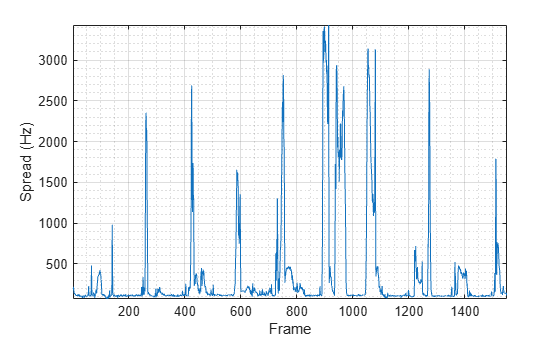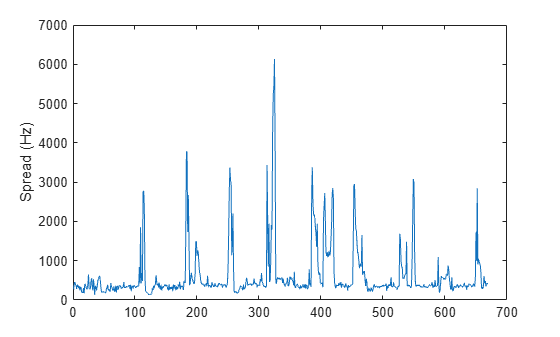Spectral spread for audio signals and auditory spectrograms

## Syntax

``spread = spectralSpread(x,f)``
``spread = spectralSpread(x,f,Name,Value)``
``[spread,centroid] = spectralSpread(___)``

## Description

example

````spread = spectralSpread(x,f)` returns the spectral spread of the signal, `x`, over time. How the function interprets `x` depends on the shape of `f`.```

example

````spread = spectralSpread(x,f,Name,Value)` specifies options using one or more `Name,Value` pair arguments.```
````[spread,centroid] = spectralSpread(___)` returns the spectral centroid.```

## Examples

collapse all

Read in an audio file, calculate the spread using default parameters, and then plot the results.

```[audioIn,fs] = audioread('Counting-16-44p1-mono-15secs.wav'); spread = spectralSpread(audioIn,fs); t = linspace(0,size(audioIn,1)/fs,size(spread,1)); plot(t,spread) xlabel('Time (s)') ylabel('Spread (Hz)')```Read in an audio file and then calculate the mel spectrogram using the `melSpectrogram` function. Calculate the spread of the mel spectrums over time. Plot the results.

```[audioIn,fs] = audioread('Counting-16-44p1-mono-15secs.wav'); [s,cf,t] = melSpectrogram(audioIn,fs); spread = spectralSpread(s,cf); plot(t,spread) xlabel('Time (s)') ylabel('Spread (Hz)')````[audioIn,fs] = audioread('Counting-16-44p1-mono-15secs.wav');`

Calculate the spread of the power spectrum over time. Calculate the spread for 50 ms Hamming windows of data with 25 ms overlap. Use the range from 62.5 Hz to `fs`/2 for the spread calculation. Plot the results.

```spread = spectralSpread(audioIn,fs, ... 'Window',hamming(round(0.05*fs)), ... 'OverlapLength',round(0.025*fs), ... 'Range',[62.5,fs/2]); t = linspace(0,size(audioIn,1)/fs,size(spread,1)); plot(t,spread) xlabel('Time (s)') ylabel('Spread (Hz)')```Create a `dsp.AudioFileReader` object to read in audio data frame-by-frame. Create a `dsp.SignalSink` to log the spectral spread calculation.

```fileReader = dsp.AudioFileReader('Counting-16-44p1-mono-15secs.wav'); logger = dsp.SignalSink;```

In an audio stream loop:

1. Read in a frame of audio data.

2. Calculate the spectral spread for the frame of audio.

3. Log the spectral spread for later plotting.

To calculate the spectral spread for only a given input frame, specify a window with the same number of samples as the input, and set the overlap length to zero. Plot the logged data.

```win = hamming(fileReader.SamplesPerFrame); while ~isDone(fileReader) audioIn = fileReader(); spread = spectralSpread(audioIn,fileReader.SampleRate, ... 'Window',win, ... 'OverlapLength',0); logger(spread) end plot(logger.Buffer) ylabel('Spread (Hz)')```Use `dsp.AsyncBuffer` if

• The input to your audio stream loop has a variable samples-per-frame.

• The input to your audio stream loop has an inconsistent samples-per-frame with the analysis window of `spectralSpread`.

• You want to calculate the spectral spread for overlapped data.

Create a `dsp.AsyncBuffer` object, reset the logger, and release the file reader.

```buff = dsp.AsyncBuffer; reset(logger) release(fileReader)```

Specify that the spectral spread is calculated for 50 ms frames with a 25 ms overlap.

```fs = fileReader.SampleRate; samplesPerFrame = round(fs*0.05); samplesOverlap = round(fs*0.025); samplesPerHop = samplesPerFrame - samplesOverlap; win = hamming(samplesPerFrame); while ~isDone(fileReader) audioIn = fileReader(); write(buff,audioIn); while buff.NumUnreadSamples >= samplesPerHop audioBuffered = read(buff,samplesPerFrame,samplesOverlap); spread = spectralSpread(audioBuffered,fs, ... 'Window',win, ... 'OverlapLength',0); logger(spread) end end release(fileReader)```

Plot the logged data.

```plot(logger.Buffer) ylabel('Spread (Hz)')```## Input Arguments

collapse all

Input signal, specified as a vector, matrix, or 3-D array. How the function interprets `x` depends on the shape of `f`.

Data Types: `single` | `double`

Sample rate or frequency vector in Hz, specified as a scalar or vector, respectively. How the function interprets `x` depends on the shape of `f`:

• If `f` is a scalar, `x` is interpreted as a time-domain signal, and `f` is interpreted as the sample rate. In this case, `x` must be a real vector or matrix. If `x` is specified as a matrix, the columns are interpreted as individual channels.

• If `f` is a vector, `x` is interpreted as a frequency-domain signal, and `f` is interpreted as the frequencies, in Hz, corresponding to the rows of `x`. In this case, `x` must be a real L-by-M-by-N array, where L is the number of spectral values at given frequencies of `f`, M is the number of individual spectrums, and N is the number of channels.

• The number of rows of `x`, L, must be equal to the number of elements of `f`.

Data Types: `single` | `double`

### Name-Value Pair Arguments

Specify optional comma-separated pairs of `Name,Value` arguments. `Name` is the argument name and `Value` is the corresponding value. `Name` must appear inside quotes. You can specify several name and value pair arguments in any order as `Name1,Value1,...,NameN,ValueN`.

Example: `'Window',hamming(256)`

Note

The following name-value pair arguments apply if `x` is a time-domain signal. If `x` is a frequency-domain signal, name-value pair arguments are ignored.

Window applied in the time domain, specified as the comma-separated pair consisting of `'Window'` and a real vector. The number of elements in the vector must be in the range [1, `size(x,1)`]. The number of elements in the vector must also be greater than `OverlapLength`.

Data Types: `single` | `double`

Number of samples overlapped between adjacent windows, specified as the comma-separated pair consisting of `'OverlapLength'` and an integer in the range [0, `size(Window,1)`).

Data Types: `single` | `double`

Number of bins used to calculate the DFT of windowed input samples, specified as the comma-separated pair consisting of `'FFTLength'` and a positive scalar integer. If unspecified, `FFTLength` defaults to the number of elements in the `Window`.

Data Types: `single` | `double`

Frequency range in Hz, specified as the comma-separated pair consisting of `'Range'` and a two-element row vector of increasing real values in the range [0, `f`/2].

Data Types: `single` | `double`

Spectrum type, specified as the comma-separated pair consisting of `'SpectrumType'` and `'power'` or `'magnitude'`:

• `'power'` –– The spectral spread is calculated for the one-sided power spectrum.

• `'magnitude'` –– The spectral spread is calculated for the one-sided magnitude spectrum.

Data Types: `char` | `string`

## Output Arguments

collapse all

Spectral spread in Hz, returned as a scalar, vector, or matrix. Each row of `centroid` corresponds to the spectral spread of a window of `x`. Each column of `spread` corresponds to an independent channel.

Spectral centroid in Hz, returned as a scalar, vector, or matrix. Each row of `centroid` corresponds to the spectral centroid of a window of `x`. Each column of `centroid` corresponds to an independent channel.

## Algorithms

The spectral spread is calculated as described in :

`$\text{spread}=\sqrt{\frac{\sum _{k={b}_{1}}^{{b}_{2}}{\left({f}_{k}-{\mu }_{1}\right)}^{2}{s}_{k}}{\sum _{k={b}_{1}}^{{b}_{2}}{s}_{k}}}$`

where

• fk is the frequency in Hz corresponding to bin k.

• sk is the spectral value at bin k.

• b1 and b2 are the band edges, in bins, over which to calculate the spectral spread.

• μ1 is the spectral centroid, calculated as described by the `spectralCentroid` function.

 Peeters, G. "A Large Set of Audio Features for Sound Description (Similarity and Classification) in the CUIDADO Project." Technical Report; IRCAM: Paris, France, 2004.

## SupportGet trial now